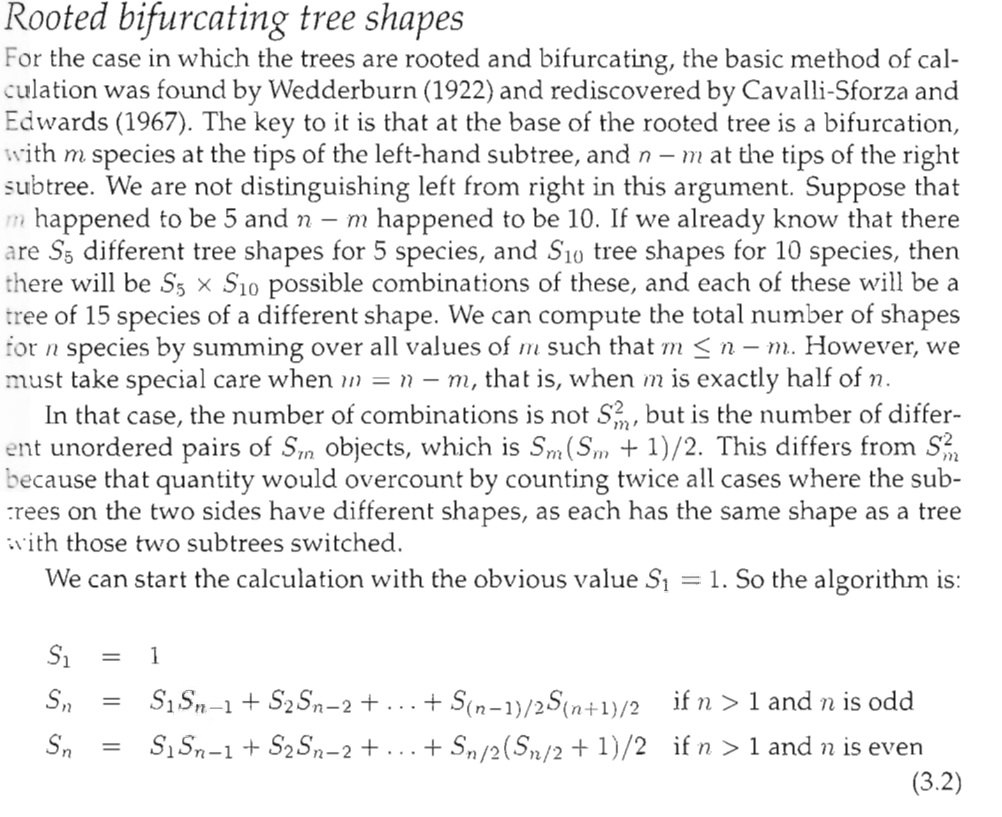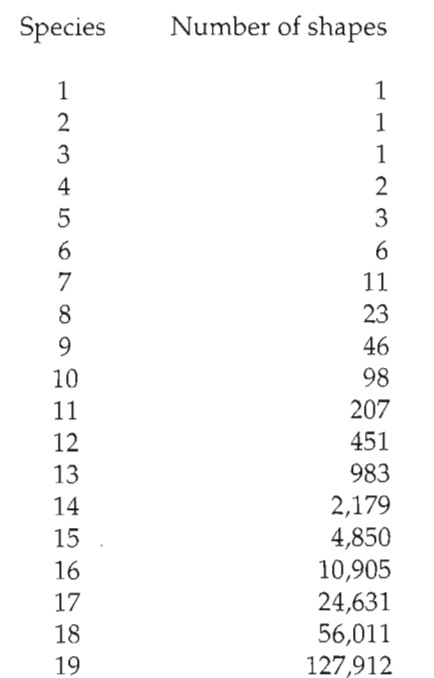How to calculate rooted bifurcating tree shapes
1
0
Entering edit mode
3.2 years ago
tractnet • 0

Hi friends!

I'm studying about phylogenetic tree shapes. Most precisely about calculate all possible rooted bifurcatingtree shapes (not labeled). A book of Felsenstein named "Inferring Phylogenies" gives an algorithm to calculate that, but I don't understand how to.

The book provides a calculation as follows:But my calculations does not match with following values table showed on the book.can anybody help me with a step by step example??

genome • 663 views
0
Entering edit mode
3.2 years ago

An R implementation would be as follows:

S <- NULL
for (n in 1:19) {
if (n == 1) {
S <- 1
} else if (n%%2 == 0) {
S <- c(S,
sum(S[seq_len((n/2)-1)] * S[(n-1):(n-((n/2)-1))]) +
S[n/2] * (S[(n/2)]+1)/2)
} else {
S <- c(S,
sum(S[seq_len((n-1)/2-1)] * S[(n-1):(n-((n-1)/2-1))]) +
S[(n-1)/2] * S[(n+1)/2])
}
}
print(S)


yielding

> print(results)
      1      1      1      2      3      6     11     23     46     98    207    451    983   2179   4850  10905
  24631  56011 127912


The key is that the summation Sn = S(1)*S(n-1) + S(2)*S(n-2) only continues to the ((n-1)/2 - 1)th term, after which the final term S((n-1)/2)*S((n+1)/2) is added (odd case). For instance, if n = 9, the ((n-1)/2 - 1)th term is S(3), and the summation would be S(1)*S(n-1) + S(2)*S(n-2) + S(3)*S(n-3) + S(4)*S((n+1)/2)